﻿

### On sets of type$(m,h)_2$ in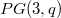$PG(3,q)$ with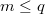$m\leq q$

#### Abstract

A set of points of$PG(3, q)$ of type$(m, h)_2$, with$m\le q$, has size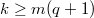$k\ge m(q+1)$. In this paper, some characterization results of some sets of type$(m,h)_2$,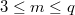$3\le m\le q$, of minimal size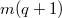$m(q+1)$ are given. Finally, sets of type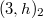$(3, h)_2$ in$PG(3, q)$ are studied.

DOI Code: 10.1285/i15900932v35n1p109

Keywords: projective space; linear code; intersection number; linear space

Full Text: PDF